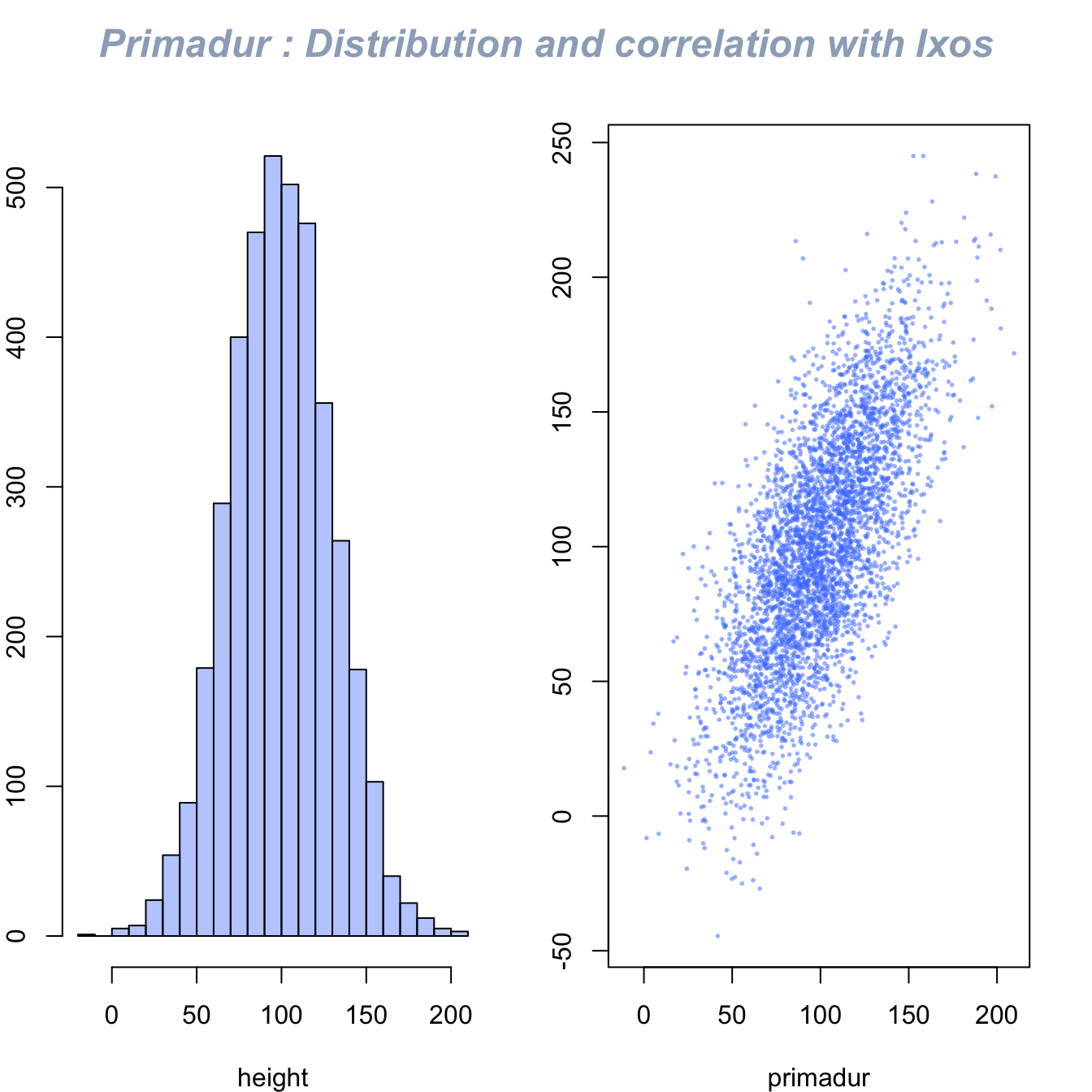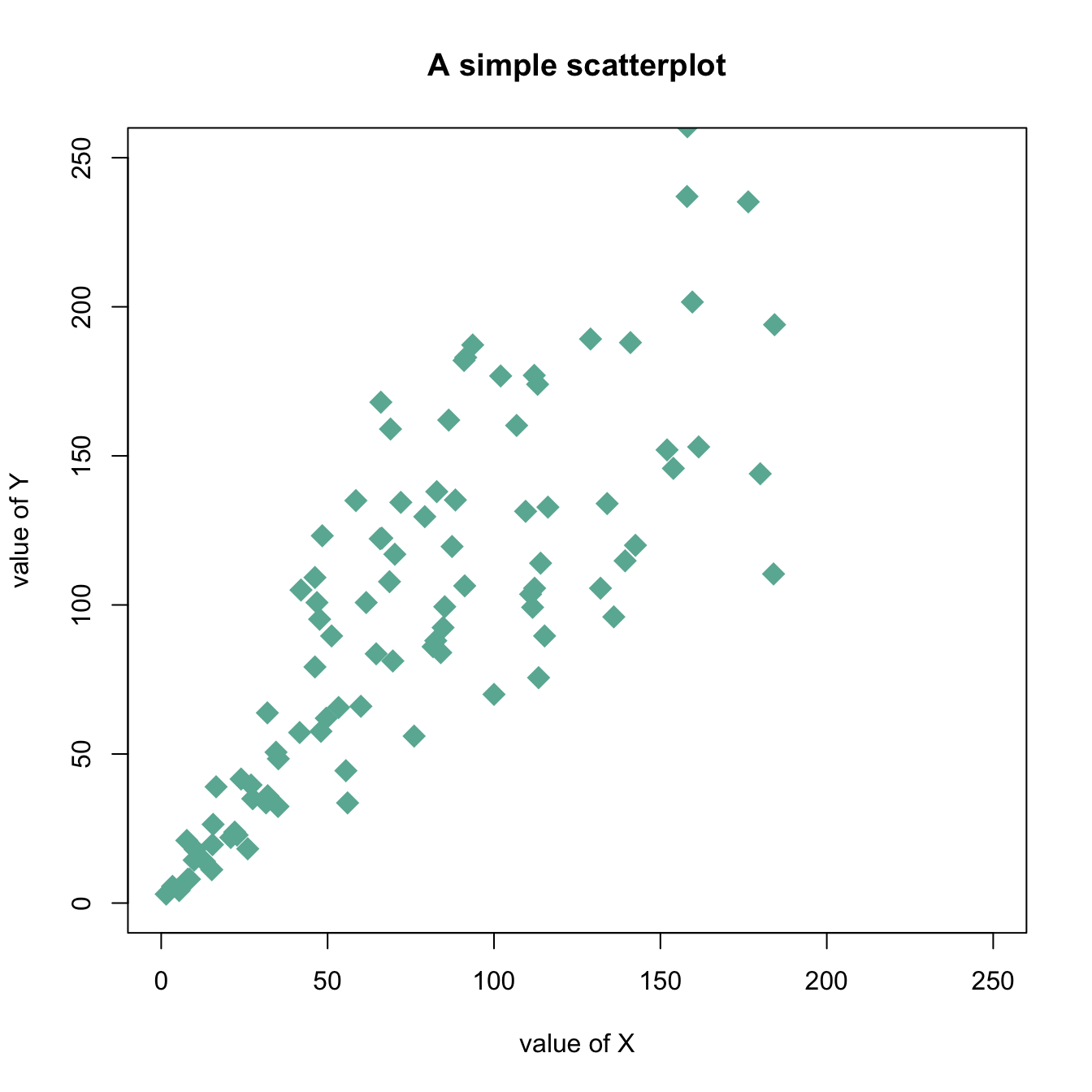# Use mtext() to write text in margin

This document describes how to use the `mtext()` function to add text in the plot margin. Usefull to add title on a multi chart.

The `mtext()` function allows to write text in one of the four margins of the current figure region or one of the outer margins of the device region.

Here, the figure is first split thanks to `par(mfrow..)`. Then, only one title is added and centered using `mtext()`.``````#Dummy data
Ixos <- rnorm(4000,100,30)
Primadur <- Ixos+rnorm(4000 , 0 , 30)

#Divide the screen in 1 line and 2 columns
par(
mfrow=c(1,2),
oma = c(0, 0, 2, 0)
)

#Make the margin around each graph a bit smaller
par(mar=c(4,2,2,2))

# Histogram and Scatterplot
hist(Ixos,  main="" , breaks=30 , col=rgb(0.3,0.5,1,0.4) , xlab="height" , ylab="nbr of plants")
plot(Ixos , Primadur,  main="" , pch=20 , cex=0.4 , col=rgb(0.3,0.5,1,0.4)  , xlab="primadur" , ylab="Ixos" )

#And I add only ONE title :
mtext("Primadur : Distribution and correlation with Ixos", outer = TRUE, cex = 1.5, font=4, col=rgb(0.1,0.3,0.5,0.5) )``````

# Customizations

Here is a description of the most common customization:

• `cex`: circle size
• `xlim` and `ylim`: limits of the X and Y axis
• `pch`: shape of markers. See all here.
• `xlab` and `ylab`: X and Y axis labels
• `col`: marker color
• `main`: chart title``````# Create data
data = data.frame(
x=seq(1:100) + 0.1*seq(1:100)*sample(c(1:10) , 100 , replace=T),
y=seq(1:100) + 0.2*seq(1:100)*sample(c(1:10) , 100 , replace=T)
)

# Basic scatterplot
plot(data\$x, data\$y,
xlim=c(0,250) , ylim=c(0,250),
pch=18,
cex=2,
col="#69b3a2",
xlab="value of X", ylab="value of Y",
main="A simple scatterplot"
)``````

Related chart types

## Contact

This document is a work by Yan Holtz. Any feedback is highly encouraged. You can fill an issue on Github, drop me a message on Twitter, or send an email pasting yan.holtz.data with gmail.com.